Searching CrossRef for 10.1016/j.neuroimage.2015.02.041...

Searching CrossRef for 10.1016/j.neuroimage.2010.10.070...

Searching CrossRef for 10.1002/nbm.3056...

Searching CrossRef for 10.1002/mrm.23000...

Searching CrossRef for 10.1016/j.neuroimage.2010.01.108...

Searching CrossRef for 10.1561/2200000016...

Searching CrossRef for 10.1364/OL.28.001194...

Searching CrossRef for 10.1109/TMI.2010.2093536...

TGV-Regularized Single-Step Quantitative Susceptibility Mapping
Itthi Chatnuntawech1, Patrick McDaniel1, Stephen F. Cauley2,3, Borjan A. Gagoski3,4, Christian Langkammer5, Adrian Martin6, Ellen Grant3,4, Lawrence L. Wald2,3,7, Kawin Setsompop2,3, Elfar Adalsteinsson1,7,8, and Berkin Bilgic2

1Electrical Engineering and Computer Science, Massachusetts Institute of Technology, Cambridge, MA, United States, 2Department of Radiology, A. A. Martinos Center for Biomedical Imaging, Charlestown, MA, United States, 3Harvard Medical School, Boston, MA, United States, 4Fetal-Neonatal Neuroimaging & Developmental Science Center, Boston Children’s Hospital, Boston, MA, United States, 5Department of Neurology, Medical University of Graz, Graz, Austria, 6Applied Mathematics, Universidad Rey Juan Carlos, Madrid, Spain, 7Harvard-MIT Health Sciences and Technology, Cambridge, MA, United States, 8Institute for Medical Engineering and Science, Cambridge, MA, United States

### Synopsis

To directly estimate tissue magnetic susceptibility distribution from the raw phase of a gradient echo acquisition, we propose a single-step quantitative susceptibility mapping (QSM) method that benefits from its three components: (i) the single-step processing that prevents error propagation normally encountered in multiple-step QSM algorithms, (ii) multiple spherical mean value kernels that permit high fidelity background removal, and (iii) total generalized variation regularization that promotes a piecewise-smooth solution without staircasing artifacts. A fast solver for the proposed method, which enables simple analytical solutions for all of the optimization steps, is also developed. Improved image quality over conventional QSM algorithms is demonstrated using the SNR-efficient Wave-CAIPI and 3D-EPI acquisitions.

### Purpose

Quantitative Susceptibility Mapping (QSM) estimates the underlying tissue magnetic susceptibility $\chi$$\chi$ from the gradient echo (GRE) phase signal. Conventional QSM algorithms involve sequential solution of multiple ill-posed post-processing steps. Recently, QSM algorithms that directly relate the unprocessed GRE phase to the unknown $\chi$$\chi$ have been proposed to improve the susceptibility estimation by preventing error propagation through successive operations in conventional QSM1-5. In this work, we propose a QSM model that combines the benefits from using a single-step processing, total generalized variation regularization (TGV)6, and multiple spherical mean value (SMV) kernels7-9. A fast solver for the proposed single-step model, which enables simple analytical solutions for all of the optimization steps, is also developed. We demonstrate the performance of the proposed method on three in vivo experiments acquired using the rapid 3D-EPI10 and Wave-CAIPI trajectories11. Herein, we also demonstrate the multi-echo capability of Wave-CAIPI sequence for the first time, and introduce an automated, phase-sensitive coil sensitivity estimation scheme.

### Methods

The underlying $\chi$$\chi$ is estimated from the unprocessed GRE phase $\varphi$$\phi$ by solving the following optimization problem

$\underset{\chi }{min}\frac{1}{2}\sum _{i}\parallel {M}_{i}\left({h}_{i}\ast d\ast \chi \right)-{M}_{i}\left({h}_{i}\ast \mathrm{\Psi }\left(\varphi \right)\right){\parallel }_{2}^{2}+TG{V}_{\alpha }^{2}\left(\chi \right)$

where $\mathrm{\Psi }$$\Psi$ is the Laplacian unwrapping operator, $d$$d$ is the spatial dipole kernel, and ${h}_{i}$$h_{i}$ is the SMV kernel for the reliable phase region indicated by the mask ${M}_{i}$$M_{i}$. Large SMV kernels are used to avoid residual error amplification, whereas smaller SMV kernels are used near the object boundary to prevent eliminating cortical phase information7-9. ${TGV}_{\alpha }^{2}$${TGV}^2_\alpha$ is the second-order TGV.

The first term combines phase unwrapping, background phase removal using multiple SMV kernels, and dipole inversion. The second term imposes prior information by promoting a piecewise-smooth solution. By using the convolution theorem and discrete form of $TG{V}_{\alpha }^{2}$$TGV^2_\alpha$, we obtain

$\underset{\chi ,v}{min}\frac{1}{2}\sum _{i}\parallel {M}_{i}{F}^{-1}{H}_{i}DF\chi -{M}_{i}{F}^{-1}{H}_{i}F\mathrm{\Psi }\left(\varphi \right){\parallel }_{2}^{2}+{\alpha }_{1}\parallel G\chi -v{\parallel }_{1}+{\alpha }_{0}\parallel \mathcal{E}\left(v\right){\parallel }_{1}$

where $F$$F$ is the discrete Fourier transform, $D=1/3-{k}_{z}^{2}/\left({k}_{x}^{2}+{k}_{y}^{2}+{k}_{z}^{2}\right)$$D=1/3-k_z^2/(k_x^2+k_y^2+k_z^2)$ is the dipole kernel in k-space, ${H}_{i}$$H_{i}$ is ${h}_{i}$$h_{i}$ in k-space, $G$$G$ is the 3D gradient operator, and $\mathcal{E}$$\mathcal{E}$ is a symmetrized derivative. By introducing auxiliary variables ${z}_{0}$$z_0$, ${z}_{1}$$z_1$ , and ${z}_{2}$$z_2$ with consensus constraints (${z}_{0}=\mathcal{E}\left(v\right)$$z_0=\mathcal{E}(v)$, ${z}_{1}=G\chi -v$$z_1=G\chi-v$, and ${z}_{2,i}={H}_{i}DF\chi$$z_{2,i}=H_iDF\chi$), applying alternating direction method of multipliers (ADMM)12, and exploiting the circulant structure of the matrices, analytical solutions are developed for all of the optimization steps.

Experiments

Using in vivo 3D EPI, Multi-Echo Wave-CAIPI at 3T, and high-resolution Wave-CAIPI data at 7T, we compared the performances of four QSM methods:

(i) SMV background filtering, followed by the dipole inversion with ${l}_{2}$$l_2$-quadratic smoothing regularization defined as $\parallel G\chi {\parallel }_{2}^{2}$$\parallel G\chi\parallel_2^2$, (V-SHARP L2),

(ii) SMV background filtering, followed by TGV-regularized dipole inversion (V-SHARP TGV),

(iii) Single-step QSM with ${l}_{2}$$l_2$-quadratic smoothing regularization (Single-Step L2), and

(iv) Single-step QSM with TGV regularization (Proposed: Single-Step TGV).

This work also introduces the multi-echo extension of Wave-CAIPI that fully utilizes the repetition time to sample multiple echoes, which are combined to improve both magnitude and phase signal-to-noise ratios (Figure 1). In addition, we develop a novel fully-automated coil sensitivity estimation based on ESPIRiT13 and virtual body coil concept14 (described in Figure 2).

For all the reconstruction methods, the GRE phase was unwrapped using Laplacian-based phase unwrapping15-16. For V-SHARP L2 and Single-Step L2, the MATLAB pcg function was used as a solver with the residual tolerance of 0.1%, and 14 SMV kernels were used with their radii ranging from 1 to 14 voxels. For V-SHARP TGV and Single-Step TGV, ADMM was used with the stopping criterion that iterations were terminated when the change in the solution between consecutive iterations was less than 1%, and 5 SMV kernels were used with their radii ranging from 1 to 5 voxels.

### Results

Figure 3 through 5 display the reconstructed susceptibility maps from the total field map using the different QSM algorithms. The proposed method had lowest level of dipole artifacts and background phase contamination while preserving the edges.

### Discussion and Conclusion

The proposed method better mitigated the dipole artifacts and improved homogeneity of image contrast compared to other QSM methods evaluated thanks to its three main components: (i) the single-step processing that prevents error propagation through successive operations, (ii) multiple SMV kernels that permit high fidelity background removal while retaining the cortical phase information, and (iii) TGV regularization that promotes a piecewise-smooth solution without staircasing artifacts. The reconstruction time was minimized by using ADMM with variable splitting in both the data and regularization terms17 and exploiting the structure of the matrices. We also introduced an automated sensitivity estimation scheme that relies on a rapid calibration acquisition for phase-sensitive coil combination. This permitted high quality parallel imaging with Wave-CAIPI, which was extended to acquire multiple echoes for improved SNR. Combination of Multi-Echo Wave-CAIPI and single-step QSM with TGV regularization thus enabled high-quality susceptibility mapping with efficient acquisition and reconstruction.

### Acknowledgements

NIH R01EB017219, P41EB015896, 1U01MH093765, R24MH106096, 1R01EB017337-01

### References

1. Bilgic B, Langkammer C, Wald LL, Setsompop K. Single-Step QSM with Fast Reconstruction. In: Third International Workshop on MRI Phase Contrast & Quantitative Susceptibility Mapping, Durham, NC, USA, 2014.

2. Bredies K, Ropele S, Poser B a, Barth M, Langkammer C. Single-Step Quantitative Susceptibility Mapping using Total Generalized Variation and 3D EPI. In: Proceedings of the 22nd Annual Meeting of ISMRM, Milan, Italy, 2014;62:604.

3. Langkammer C, Bredies K, Poser BA, Barth M, Reishofer G, Fan AP, Bilgic B, Fazekas F, Mainero C, Ropele S. Fast quantitative susceptibility mapping using 3D EPI and total generalized variation. Neuroimage 2015;111:622–30. doi: 10.1016/j.neuroimage.2015.02.041.

4. Liu T, Zhou D, Spincemaille P, Yi W. Differential approach to quantitative susceptibility mapping without background field removal. In: Proceedings of the 22nd Annual Meeting of ISMRM, Milan, Italy, 2014. p. 597.

5. Sharma S, Hernando D, Horng D, Reeder S. A joint background field removal and dipole deconvolution approach for quantitative susceptibility mapping in the liver. In: Proceedings of the 22nd Annual Meeting of ISMRM, Milan, Italy, 2014. p. 606.

6. Bredies K, Kunisch K, Pock T. Total Generalized Variation. SIAM J. Imaging Sci. 2010;3:492–526.

7. Schweser F, Deistung A, Lehr BW, Reichenbach JR. Quantitative imaging of intrinsic magnetic tissue properties using MRI signal phase: An approach to in vivo brain iron metabolism? Neuroimage 2011;54:2789–2807. doi: 10.1016/j.neuroimage.2010.10.070.

8. Li W, Avram A V., Wu B, Xiao X, Liu C. Integrated Laplacian-based phase unwrapping and background phase removal for quantitative susceptibility mapping. NMR Biomed. 2014;27:219–227. doi: 10.1002/nbm.3056.

9. Wu B, Li W, Guidon A, Liu C. Whole brain susceptibility mapping using compressed sensing. Magn. Reson. Med. 2012;67:137–47. doi: 10.1002/mrm.23000.

10. Poser B a., Koopmans PJ, Witzel T, Wald LL, Barth M. Three dimensional echo-planar imaging at 7 Tesla. Neuroimage 2010;51:261–266. doi: 10.1016/j.neuroimage.2010.01.108.

11. Bilgic B, Gagoski BA, Cauley SF, Fan AP, Polimeni JR, Grant PE, Wald LL, Setsompop K. Wave-CAIPI for highly accelerated 3D imaging. Magn. Reson. Med. 2015;73:2152–2162.

12. Boyd S, Parikh N, Chu E, Peleato B, Eckstein J. Distributed Optimization and Statistical Learning via the Alternating Direction Method of Multipliers. Found. Trends® Mach. Learn. 2010;3:1–122. doi: 10.1561/2200000016.

13. Uecker M, Lai P, Murphy M, Virtue P, Elad M, Pauly JM, Vasanawala S, Lustig M. ESPIRiT—an eigenvalue approach to autocalibrating parallel MRI: Where SENSE meets GRAPPA. Magn. Reson. Med. 2014;71:990–1001.

14. Buehrer M, Boesiger P, Kozerke S. Virtual Body Coil Calibration for Phased-Array Imaging. In: Proceedings of the 17th Annual Meeting of ISMRM, Honolulu, Hawaii, USA, 2009. p. 760.

15. Li W, Wu B, Liu C. Quantitative susceptibility mapping of human brain reflects spatial variation in tissue composition. Neuroimage 2011;55:1645–1656.

16. Schofield MA, Zhu Y. Fast phase unwrapping algorithm for interferometric applications. Opt. Lett. 2003;28:1194. doi: 10.1364/OL.28.001194.

17. Ramani S, Fessler JA. Parallel MR Image Reconstruction Using Augmented Lagrangian Methods. IEEE Trans. Med. Imaging 2011;30:694–706. doi: 10.1109/TMI.2010.2093536.

### Figures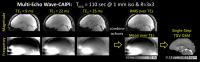Figure 1: Multi-Echo Wave-CAIPI. The magnitude images from the three echoes are merged with root-mean-square combination, while the phase images are unwrapped, normalized by their TEs, and then averaged for improved SNR.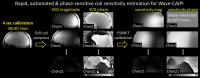Figure 2: The phase of the coil sensitivities obtained from ESPIRiT is conventionally taken to be relative to the reference channel. If phase singularities are present in the reference channel, they will propagate to the other channels. To address this, we employ the virtual body coil concept. We apply SVD coil compression to a calibration acquisition and then input the dominant singular vector, which is observed to have slowly-varying phase, to ESPIRiT as the reference channel.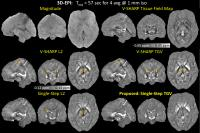Figure 3: 3D-EPI at 3T with field-of-view (FOV) = 230 mm x 230 mm x 176 mm, spatial resolution = 1 mm isotropic, TR = 69 ms, echo time (TE) = 21 ms, acceleration factor (R) = 4, number of receiver coils = 32, and total acquisition time = 57 s. The proposed Single-Step TGV method successfully mitigated the dipole artifacts (orange arrows) and gave better image contrast compared to the other methods.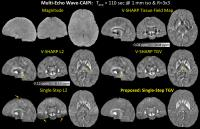Figure 4: Multi-Echo Wave-CAIPI at 3T with FOV = 256 mm x 192 mm x 120 mm, spatial resolution = 1 mm isotropic, TR = 43 ms, TEs = 9/22/35 ms, R = 3x3, number of receiver coils = 32, and total acquisition time = 110 s. The proposed method had the lowest level of dipole artifacts and background phase contamination (orange arrows).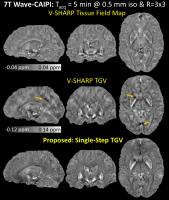Figure 5: Wave-CAIPI at 7T with FOV = 224 mm x 185 mm x 120 mm, spatial resolution = 0.5 mm isotropic, TR = 30 ms, TE = 20 ms, R = 3x3, number of receiver coils = 31, and total acquisition time = 5 min. The proposed method had lower level of dipole artifacts and background phase contamination (orange arrows) than V-SHARP TGV.

Proc. Intl. Soc. Mag. Reson. Med. 24 (2016)
0869